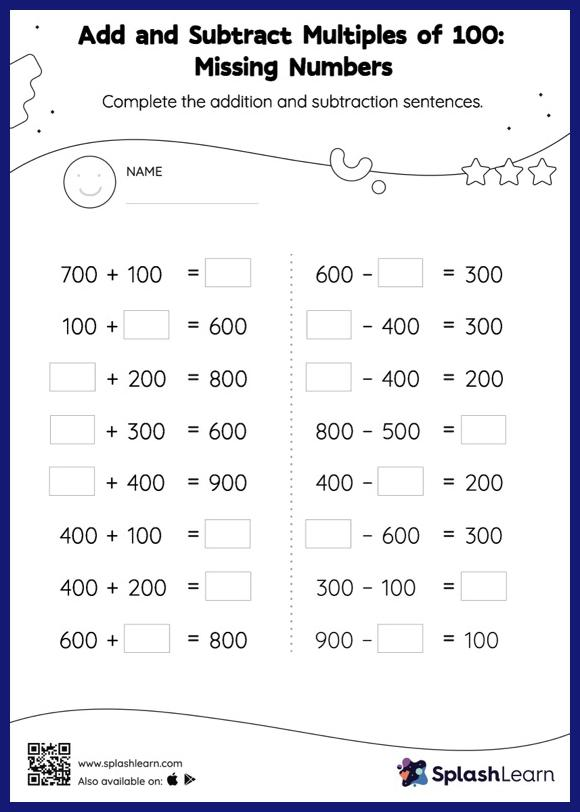# Add and Subtract Multiples of 100: Missing Numbers Worksheet

Home > Add and Subtract Multiples of 100: Missing NumbersAdd and subtract multiples of 100 worksheet helps students develop proficiency with addition and subtraction. In this worksheet, students practice solving problems written in the horizontal format. How numbers are laid out in a problem affects the method a student employs to solve it. Therefore to develop actual fluency and mastery of multiple strategies, students must practice different formats.